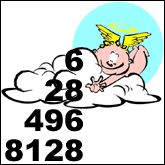# Perfect NumbersSome numbers are more special than others. According to Pythagoras (569 BC - 475 BC) and Euclid (325 BC - 265 BC), some are so special that they called them mystical or perfect numbers. The first perfect number is 6; the second is 28. The Greeks knew of two more: 496 and 8,128. Can you see a pattern? Try figuring out what is so special about these four numbers before you continue reading.

Well, the definition of a perfect number is: any number that is equal to the sum of its divisors (numbers that will divide into it without leaving a remainder). Therefore you can see that: 6 = 1 + 2 + 3; 28 = 1 + 2 + 4 + 7 + 14; and so on. Simple, right? How about the fifth perfect number? Can you come up with it? Don't even try … just continue reading.

It took mathematicians about 1,500 years (1536, Hudalrichus Regius) to discover the fifth perfect number: 33,550,336. The greatest contributions to future discoveries of perfect numbers were offered by French mathematicians Fermat and Mersenne, during early 1600s, when they devised a useful formula for finding perfect numbers. Many mathematicians have contributed since, and today we know of 39 perfect numbers. As you may guess, discoveries of new perfect numbers have become more frequent with the help of computers. The largest one has more than 4 millions digits, and was discovered in 2001. It turns out that all the perfect numbers discovered so far are even. Will we ever discover an odd perfect number? Will we discover an underlining mathematical law that prohibits this? Live and learn!

Anton Skorucak, MSAnton Skorucak is a founder and publisher of ScienceIQ.com. Anton Skorucak has a Master of Science (MS) degree in physics from the University of Southern California, Los Angeles, California and a B.Sc. in physics with a minor in material science from the McMaster University, Canada. He is the president and creator of PhysLink.com, a comprehensive physics and astronomy online education, research and reference web site.

### Science Articles: